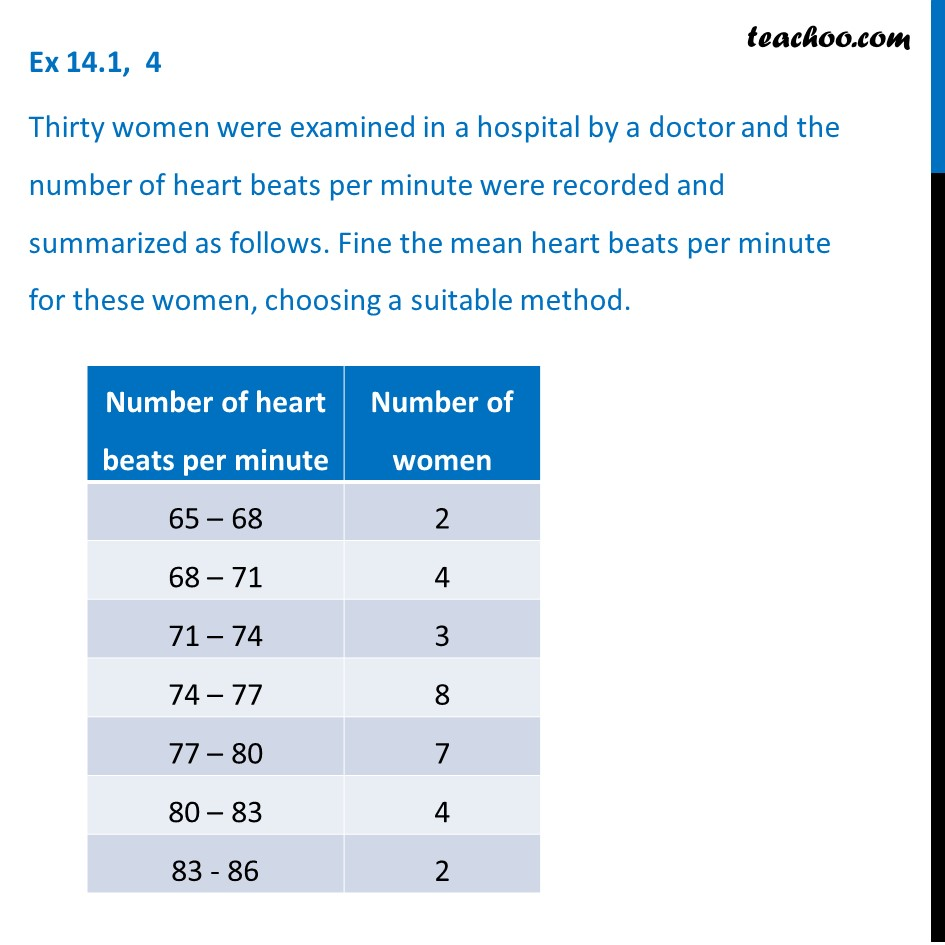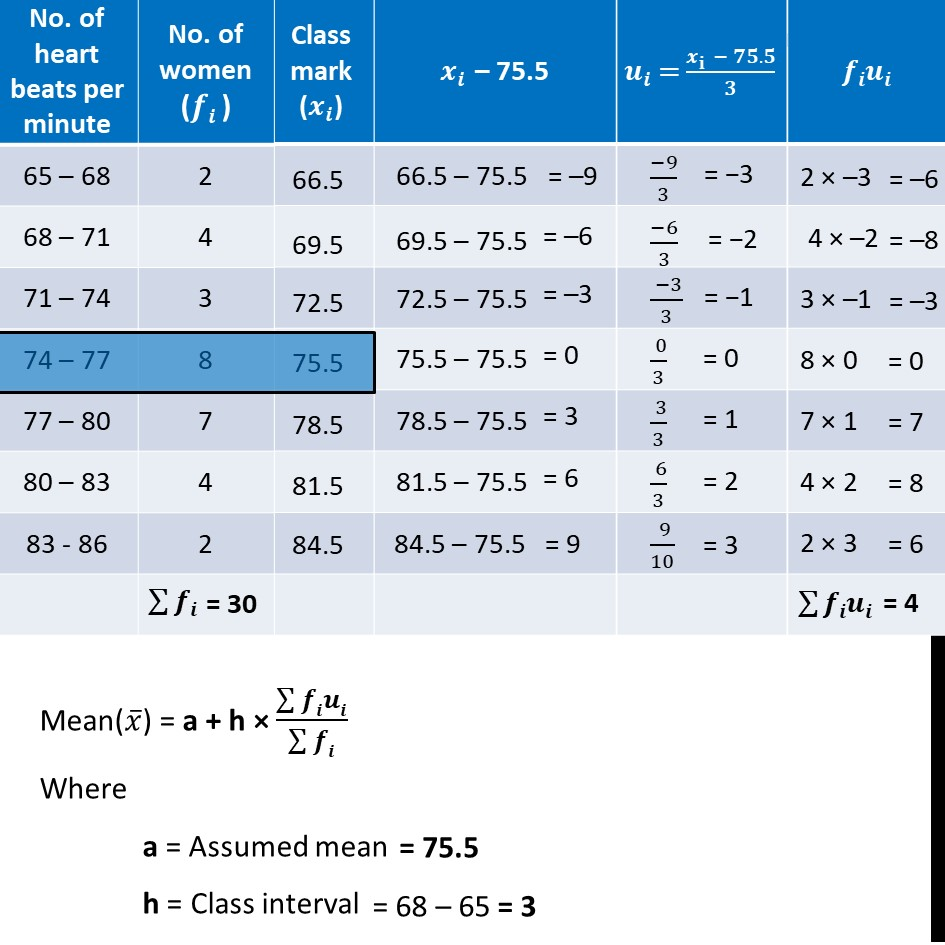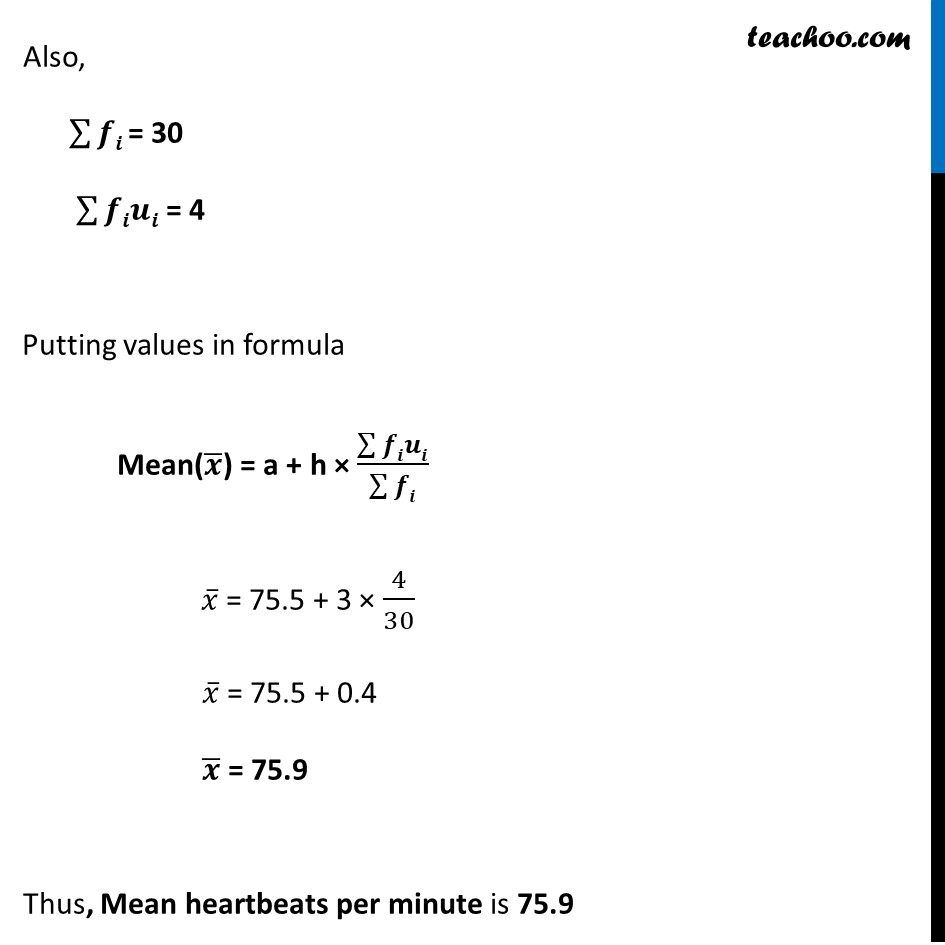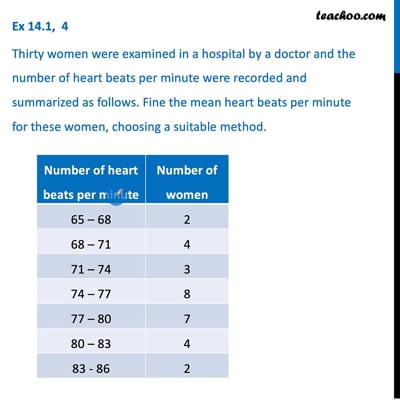Mean

Chapter 14 Class 10 Statistics
Concept wiseThis video is only available for Teachoo black users

Introducing your new favourite teacher - Teachoo Black, at only ₹83 per month

### Transcript

Ex 14.1, 4 Thirty women were examined in a hospital by a doctor and the number of heart beats per minute were recorded and summarized as follows. Fine the mean heart beats per minute for these women, choosing a suitable method. Mean(𝑥 ̅) = a + h × (∑▒𝒇𝒊𝒖𝒊)/(∑▒𝒇𝒊) Where a = Assumed mean h = Class interval = 75.5 = 68 – 65 = 3 Also, ∑▒𝒇𝒊 = 30 ∑▒𝒇𝒊𝒖𝒊 = 4 Putting values in formula Mean(𝒙 ̅) = a + h × (∑▒𝒇𝒊𝒖𝒊)/(∑▒𝒇𝒊) 𝑥 ̅ = 75.5 + 3 × 4/30 𝑥 ̅ = 75.5 + 0.4 𝒙 ̅ = 75.9 Thus, Mean heartbeats per minute is 75.9